It looks like you're using Internet Explorer 11 or older. This website works best with modern browsers such as the latest versions of Chrome, Firefox, Safari, and Edge. If you continue with this browser, you may see unexpected results.# Chemistry Lab Resources (for CHM 1XX and 2XX Labs)

Here you can find tips about organizing your lab notebook, how to effectively create graphs and table for lab reports, places to locate protocols and property information, and how to properly cite resources.

## Stoichiometry

Stoichiometry is simply the math behind chemistry. Given enough information, one can use stoichiometry to calculate masses, moles, and percents within a chemical equation.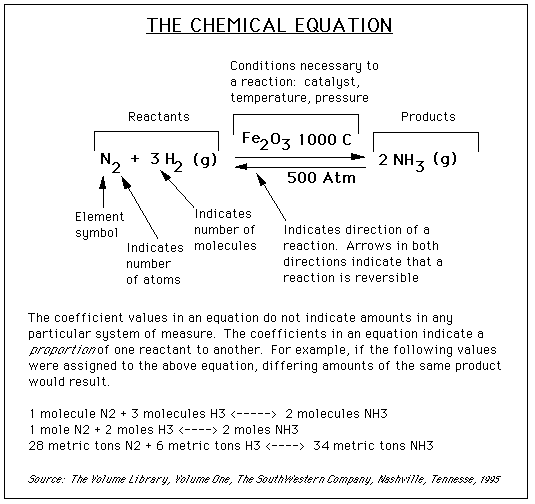##### Moles and Avogadro

A mole simply represents Avogadro's number (6.022 x 1023) of molecules. A mole is similar to a term like a dozen. If you have a dozen carrots, you have twelve of them. Similarly, if you have a mole of carrots, you have 6.022 x 1023 carrots. In the equation above there are no numbers in front of the terms, so each coefficient is assumed to be one (1). Thus, you have the same number of moles of AgNO3, NaCl, AgCl, NaNO3.

Converting between moles and grams of a substance is often important. This conversion can be easily done when the atomic and/or molecular mass of the substance(s) are known. Given the atomic or molecular mass of a substance, that mass in grams makes a mole of the substance. For example, calcium has an atomic mass of 40 atomic mass units. So, 40 grams of calcium makes one mole, 80 grams makes two moles, etc.

##### Example

Molar Mass of Ca = 40.08 (From the Periodic Table)

How many moles of Ca are in 4.50 grams of Ca?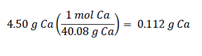Citation: Department of Chemistry at UNC Chapel Hill at http://www.shodor.org/unchem/basic/stoic/

## Limiting Reagents

Sometimes when reactions occur between two or more substances, one reactant runs out before the other. That is called the "limiting reagent". Often, it is necessary to identify the limiting reagent in a problem.
Example: A chemist only has 6.0 grams of C2H2 and an unlimited supply of oxygen and he desires to produce as much CO2 as possible. If she uses the equation below, how much oxygen should she add to the reaction?

2C2H2(g) + 5O2(g) ---> 4CO2(g) + 2 H2O(l)

To solve this problem, it is necessary to determine how much oxygen should be added if all of the reactants were used up (this is the way to produce the maximum amount of CO2).

First, we calculate the number of moles of C2H2 in 6.0 g of C2H2. To be able to calculate the moles we need to look at a periodic table and see that 1 mole of C weighs 12.0 g and H weighs 1.0 g. Therefore we know that 1 mole of C2H2 weighs 26 g (2 × 12 grams + 2 × 1 gram).

 6.0 g C2H2 x 1 mol C2H2(24.0 + 2.0)g C2H2 = 0.25 mol C2H2

Then, because there are five (5) molecules of oxygen to every two (2) molecules of C2H2, we need to multiply the result by 5/2 to get the total molecules of oxygen. Then we convert to grams to find the amount of oxygen that needs to be added:

 0.25 mol C2H2 x 5 mol O22 mol C2H2 x 32.0 g O21 mol O2 = 20 g O2

Citation: Department of Chemistry at UNC Chapel Hill at http://www.shodor.org/unchem/basic/stoic/

## Concentrations of Solutions

### Percent Composition (by mass)

We can consider percent by mass (or weight percent, as it is sometimes called) in two ways:

• The parts of solute per 100 parts of solution.
• The fraction of a solute in a solution multiplied by 100.

We need two pieces of information to calculate the percent by mass of a solute in a solution:

• The mass of the solute in the solution.
• The mass of the solution.

Use the following equation to calculate percent by mass: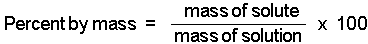### Molarity

Molarity tells us the number of moles of solute in exactly one liter of a solution. (Note that molarity is spelled with an "r" and is represented by a capital M.)

We need two pieces of information to calculate the molarity of a solute in a solution:

• The moles of solute present in the solution.
• The volume of solution (in liters) containing the solute.

To calculate molarity we use the equation: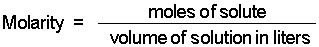## Chemical Equilibrium

Writing Expressions for Kc

The general equilibrium expression for a reaction:is written as:The brackets "[ ]" represent the concentration of the species (moles per liter or molarity). "a, b, c, and d" represent the coefficients used to balance the equation. The "c" in Kc indicates that the value of K is determined using the concentrations of each species.

There are two cases when a species is not shown in the equilibrium expression:

• when it is a solid
• when it is a pure liquid or solvent

Writing Expressions for Kp

When one or more of the species in a system exists in the gaseous phase, the partial pressure of that species can be used in the equilibrium expression  Dissolved species are still expressed as moles per liter (molarity).

Examples

Examples of equilibrium expressions Kc for a variety of equilibrium systems follow. When one or more gaseous substances are involved, the Kp expression is also given.

•   The production of ammonia from nitrogen and hydrogen gases.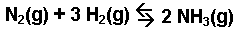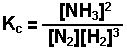• The thermal decomposition of calcium carbonate.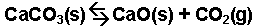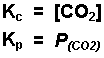• The oxidation-reduction reaction occuring between iron(III) chloride and tin(II) chloride.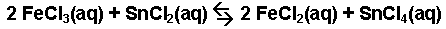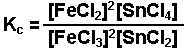## Chemical Equilibrium

How to Make an ICE Chart

In making an ICE chart the following items should be noted:

• Express all quantities in terms of MOLARITY (moles per liter).  (If using Kp, gaseous species must be expressed in appropriate pressure units.)
• Use initial quantities when calculating the reaction quotient, Q, to determine the direction the reaction shifts to establish equilibrium.
• Use equilibrium quantities in calculations involving the equilibrium constant, K.
• The change in each quantity must be in agreement with the reaction stoichiometry.
• Read each problem carefully to identify what quantities are given, including their unit of measure, and to identify what is unknown.
• Clearly define the change you choose to be represented by "x."  Define all other unknown changes in terms of this change.

The following is a "how to" make an ICE chart using the example to illustrate the process.

Example:  A mixture consisting initially of 3.00 moles NH3, 2.00 moles of N2, and 5.00 moles of H2, in a 5.00 L container was heated to 900 K, and allowed to reach equilibrium.  Determine the equilibrium concentration for each species present in the equilibrium mixture.

2 NH3(g)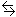N2(g) + 3 H2(g)    Kc = 0.0076 @ 900 K

• Convert the initial quantities to molarities as shown for NH3.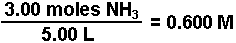• Create a chart as illustrated below and enter in the known quantities.

 NH3 N2 H2 Initial Concentration (M) 0.600 0.400 1.00 Change in Conc. (M) Equilibrium Conc. (M)
• Calculate Qc and compare to Kcto determine the direction the reaction will proceed.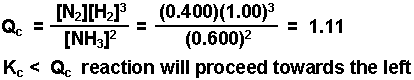• Assign a variable "x" that represents the change in the amount of one of the species.  The species with the lowest coefficient in the balanced equation usually is the easiest to handle when it comes to doing the math. Here let "x" = change in the amount of N2.
• Determine the change in all the other species in terms of "x."  Remember the change must be in agreement with the stoichiometry of the balanced equation, in this case 2:1:3.  Since the reaction goes in the reverse direction the concentrations of N2 and H2 gases will decrease (note the negative sign) and that of NH3will increase.  Put these quantities into the chart (shown in red).

 NH3 N2 H2 Initial Concentration (M) 0.600 0.400 1.00 Change in Conc. (M) + 2 x - x - 3 x Equilibrium Conc. (M)
• Express the equilibrium concentrations in terms of "x" and the initial amounts (shown in green).

 NH3 N2 H2 Initial Concentration (M) 0.600 0.400 1.00 Change in Conc. (M) + 2 x - x - 3 x Equilibrium Conc. (M) 0.600 + 2 x 0.400 - x 1.00 - 3 x
• Substitute the expressions for the equilibrium concentration into the expression for the equilibrium constant and solve for "x."  Once x is known, the equilibrium concentration for each species can be calculated.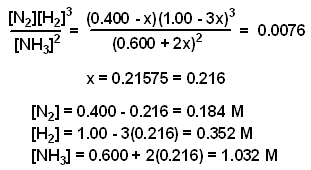The value of x was determined using the method of successive approximations.

## Kinetics

Determining the Average Rate from Change in Concentration over a Time Period

We calculate the average rate of a reaction over a time interval by dividing the change in concentration over that time period by the time interval. For the change in concentration of a reactant, the equation, where the brackets mean "concentration of", is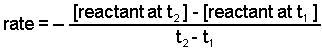Note: We use the minus sign before the ratio in the previous equation because a rate is a positive number. We do not need the minus sign when calculating average rates from products.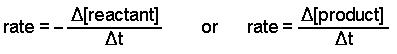Determining the Instantaneous Rate from a Plot of Concentration Versus Time

An instantaneous rate is the rate at some instant in time. An instantaneous rate is a differential rate: -d[reactant]/dt or d[product]/dt.

We determine an instantaneous rate at time t:

• by calculating the negative of the slope of the curve of concentration of a reactant versus time at time t.

• by calculating the slope of the curve of concentration of a product versus time at time t.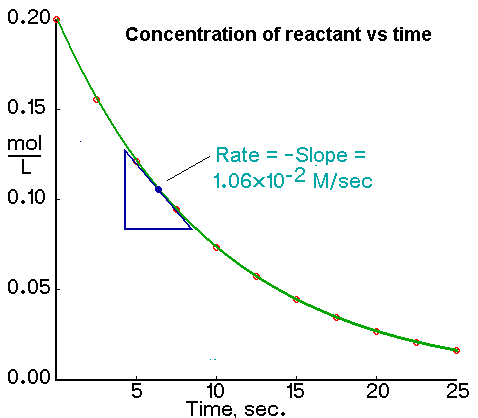Determining the Initial Rate from a Plot of Concentration Versus Time

The initial rate of a reaction is the instantaneous rate at the start of the reaction (i.e., when t = 0). The initial rate is equal to the negative of the slope of the curve of reactant concentration versus time at t = 0.

# Calorimetry

If we run an exothermic reaction in solution in a calorimeter, the heat produced by the reaction is trapped in the calorimeter and increases the temperature of the solution. If we run an endothermic reaction, the heat required by the reaction is removed from the solution and the temperature of the solution decreases.We can calculate the amount of heat absorbed by the solution or the amount of heat removed from the solution with the following equation: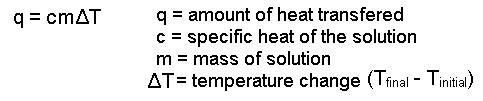Note:

• When heat is absorbed by the solution, q for the solution has a positive value. This means that the reaction produces heat for the solution to absorb and q for the reactionis negative.

• When heat is absorbed from the solution q for the solution has a negative value. This means that the reaction absorbs heat fron the solution, the reaction is endothermic, and q for the reaction is positive.